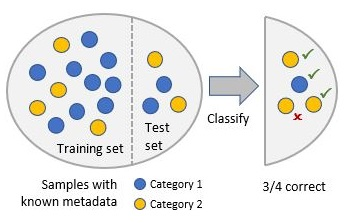﻿ K-fold cross-validation# K-fold cross-validationSee also
Machine learning
Random forests
OTU importance
forest_kfold command
otutab_forest_kfold command
otutab_select command

K-fold cross-validation is a machine learning strategy for assessing whether a classifier can be successfully trained on data with known categories. In OTU analysis, observations are samples and categories are specified by metadata (healthy / sick, day / night etc.).

If k-fold cross-validation reports high accuracy, this usually implies that the frequencies of some OTUs correlate with metadata states. For example, an OTU might have high frequency in a sick patient but low frequency in a healthy patient. See OTU importance for further discussion.

Samples labeled with known categories are randomly split into two subsets: a training set and a test set. The training set is used to train the classifier, which then predicts categories of samples in the test set. Accuracy is measured by comparing the predicted categories with the true categories. This process is repeated several times and the accuracy is averaged.

The "k" in k-fold usually refers both to the fraction of observations in the test set and the number of iterations. For example, with 5-fold cross-validation, 1/5th of the samples are assigned to the test set, and this is repeated 5 times.

Accuracy can be measured in many different ways. One approach is simply to count the number of correct and wrong predictions, ignoring the confidence value reported by the classifier except to assign the category with highest confidence. For a binary classifier (i.e. a classifier for exactly two categories), confidence can be included in the assessment by making a ROC curve. Many different accuracy metrics can be calculated, e.g. sensitivity, specificity, positive predictive value, Matthews' Correlation Coefficient etc.; see Wikipedia article for details.

In OTU analysis, the choice of accuracy metric and its numerical value are usually not very interesting. The key question is whether the accuracy is much better than a random guess, a little better than a random guess, or not better at all. If the accuracy is better than guessing, then there is information in the OTU frequencies, and the next step is then to figure out which OTUs are informative.